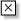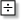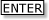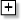Tapping into mathematics

Start this free course now. Just create an account and sign in. Enrol and complete the course for a free statement of participation or digital badge if available.

Free course

# 5.4 Percentage increases and decreases

There is no single right way of calculating percentage increases or decreases. The next examples show two different approaches to the same problems.

## Example 1

A railway season ticket from my local station to London costs (at current prices) £825. Calculate the cost of a new ticket, if prices have risen by 6%.

Method 1

To find the cost of a new ticket, you can find 6% of £825 and then add this amount on to the original cost. Remember that a given percentage is simply a fraction made up of that number divided by 100. So 6% is 6/100.

A 6% rise means a fare increase of £825 × 6/100 = £49.50

So the new cost of a season ticket is £825 + £49.50 = £874.50.

One way of doing this on the TI-84 calculator is:

• set the calculator to display 2 decimal places

• Input 8256100• Input825Method 2

This is a more elegant method. The original cost of £825 was 100%. So increasing it by 6% will give 106%. Hence work out 106% of £825. However 106% is 106/100 or 1.06. So an elegant calculation of the new fare is £825 × 1.06. Your calculator should give the new value of 825 ×1.06 = 874.50. So the cost is £874.50.

## Example 2

When I went to the local branch of a department store, I was offered 15% off the price of whatever I bought, if I signed up for the company's credit card. My purchases came to £74.64. What would the price be if I took up the company's offer?

Method 1

15% of 74.64 = 15/100 × 74.64 = 11.20 (to 2 d.p.)

So reduction is £11.20.

The new price in £ is 74.65 − 11.20 = 63.44

So I would pay £63.44.

Method 2

The original price of £74.64 is 100% and so reducing the price by 15% will give 100% – 15% or 85% of the original price. Now 85% is 85/100 or .85. So the new price in is: 0.85 × .74.64 = 63.44 (to 2 d.p.)

Hence I would pay £63.44, if I took the company's offer.

The next exercise will give you some more practice at calculating percentage increases and decreases.

## Exercise 6: Ups and downs

Practice calculating percentage changes by completing the table below. In the last line of the table you will see a line over a number: this means recurring (33.3333333333… or one third).

Original pricePercentage priceFinal price
£10077% increase£177.00
£275011% decrease£2447.50
£10018% decrease
£108035% increase
£51100% increase
£2250% increase
£333.% decrease

Original pricePercentage priceFinal price
£10077% increase£177.00
£275011% decrease£2447.50
£10018% decrease£82.00
£108035% increase£1458.00
£51100% increase£102.00
£2250% increase£7.00
£333.% decrease£2.00
MU120_4MCAL